# Find v{t) for t > 0 in the circuit of Fig. 8.98.

Question-AnswerCategory: Electrical EngineeringFind v{t) for t > 0 in the circuit of Fig. 8.98.

### Find v{t) for t > 0 in the circuit of Fig. 8.98.For Prob. 8.51.For Prob. 8.51.

Step: 1

Refer to Figure 8.98 .
Initially at, the switch is closed for a long time. The circuit has reached to steady state and the inductor acts like a short circuit, while the capacitor acts like an open circuit.
The equivalent circuit diagram is shown in Figure 1.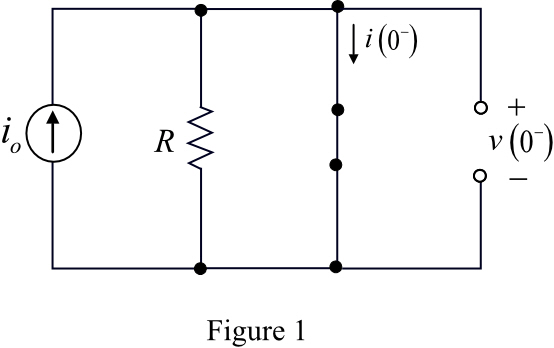Step: 2

In Figure 1, there is a short circuit across the resistor,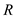. Thus, no current flows through the resistor,. Redraw the modified circuit diagram.Step: 3

From Figure 2, the initial voltage across capacitor is,The capacitor voltage does not change instantaneously.From Figure 2, the initial current through the inductor is,The inductor current does not change instantaneously.Step: 4

For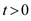, the switch is opened. The equivalent circuit diagram is shown in Figure 3.Step: 5

From Figure 3, it is a source-free parallel LC circuit.
Write the formula for neper frequency: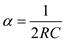In Figure 3, there is no resistance in parallel with the energy storing elements.
Therefore, the value of neper frequency is,Write the formula for resonance frequency: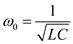From the above calculations,.
Therefore, it is an under damped system.

Step: 6

For an under damped system, the expression for voltage is,Here,Substitute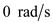forin the equation.Therefore, the expression for voltage across the capacitor is,Substitutefor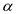in the equation.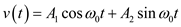…… (1)

Step: 7

Determine the value of.
Substitutefor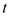in equation (1).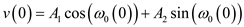Substitutefor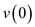in the equation.From Figure 3, the current flowing through the capacitor is,Step: 8

Differentiate the voltage expression with respect t, to determine the value of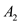.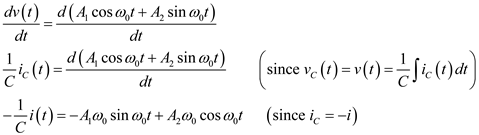Substituteforin the equation.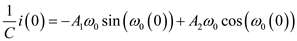Substitutefor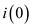in the equation.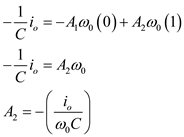Step: 9

Recall the equation (1).Substitute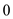for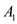and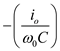forin the equation, to derive the expression for voltage across the capacitor.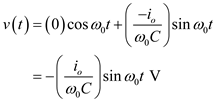Thus, the expression for voltage across the capacitor is,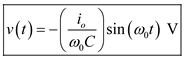.